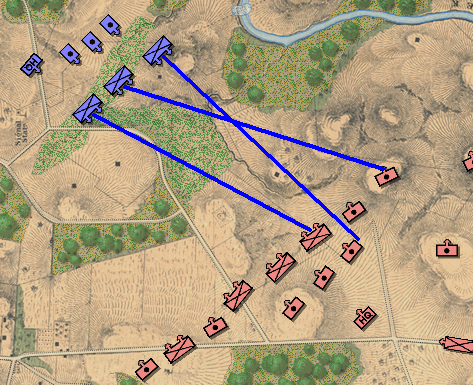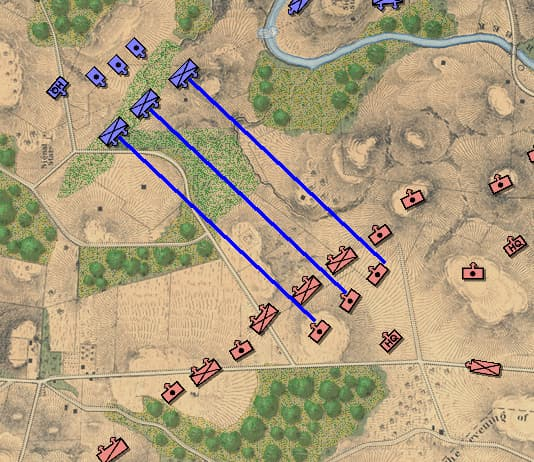# Help with intersecting lines

I need another set of eyes on this. This is code that I found somewhere that is supposed to return true if the the 2 lines intersect:

``````  public static bool DoLinesIntersect(PointI p1, PointI p2, PointI p3, PointI p4)
{
bool intersect = false;

// Get the segments' parameters.
float dx12 = p2.X - p1.X;
float dy12 = p2.Y - p1.Y;
float dx34 = p4.X - p3.X;
float dy34 = p4.Y - p3.Y;

// Solve for t1 and t2
float denominator = (dy12 * dx34 - dx12 * dy34);

float t1 = ((p1.X - p3.X) * dy34 + (p3.Y - p1.Y) * dx34) / denominator;
float t2 = ((p3.X - p1.X) * dy12 + (p1.Y - p3.Y) * dx12) / -denominator;

// The segments intersect if t1 and t2 are between 0 and 1.
if ((t1 >= 0) && (t1 <= 1) && (t2 >= 0) && (t2 <= 1))
return true;

return intersect;
}
``````

After a lot of debugging I don’t think it’s working. Can anybody confirm one way or another?
Thanks.

Edit: PS: This is graphic debugging that shows the lines of advance of 2 units are crossing. This is exactly what I’m trying to keep from happening:Not sure if this is your problem but the denominator maybe zero or close enough to zero to mess up your logic. Maybe add some code to check the denominator?

if (Math.Abs(denominator) < 0.001)
return false

I have an idea for code that could be easier:

``````public static bool DoLinesIntersect(PointI p1, PointI p2, PointI p3, PointI p4) {
return MathF.Sign((p2.X - p1.X) * (p3.Y - p1.Y) - (p2.Y - p1.Y) * (p3.X - p1.X)) != MathF.Sign((p2.X - p1.X) * (p4.Y - p1.Y) - (p2.Y - p1.Y) * (p4.X - p1.X));
}
``````

The way it works is you do the dot product using the line p1 to p2 against each p3 and p4 individually. Then you compare the result. If they are not equal then the lines intersect.

I didn’t test it thoroughly but I assume this works.

Just a note, after writing his game for him for a while, you are now fixing code he got from chatGPT.

1 Like

I certainly didn’t get this code from ChatGPT. I was looking for some line segment intersect code and found this on Stack Overflow.

I think your code is better. But, I’m still getting crossed lines of attack. I don’t know where you guys are, but I slept on the problem last night, and I’m thinking that the crossed (bad) attack line is being generated elsewhere (and not being checked for an intersection). That’s the assumption that I’m going to work with today.

Okay, figured it out. As usual, I completely over-thought the problem (I know, big surprise). I was over-thinking parallel lines (they don’t intersect) when all I had to do was calculate the offset for the other units and apply it:I really miss working with a team (and an extra pair of eyes).

Could be wrong, but the marked solution is going to work for rays and not for lines? Could make a difference as you’d get false positives

(afaik that’s what the last line in your original code does check)

What I do in such things is to prepare a little test szenario like drawing a line to mouse pointer just to make sure if the algo works as expected - if that’s proofen and the final result is still wrong, the error is elsewhere

1 Like

Just to be safe, I unchecked it as the solution. I never actually ran the code because I realized that my entire approach to the problem WAS COMPLETELY WRONG! The solution, as always, was simple. The problem, as usual, was that I over-thought it.

Check out the image, above. Once I realized it was simply a matter of calculating the offset I could easily make the other units advance parallel to the ‘lead unit’. Surprisingly, this is pretty much how 19th century land warfare worked.